This math fractions ClipArt gallery offers 14 illustrations of a circle, or pie, broken into various sized slices. These images often have math to supplement the division of the image.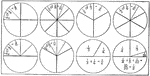### Fraction Circles

Circles showing fraction values and how to multiply and add fractions.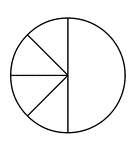### Four Eighths and One Half of a Pie Fraction

A circle subdivided into four eighths and one half.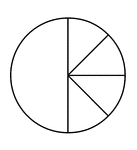### One Half and Four Eighths of a Pie Fraction

A circle subdivided into one half and four eighths.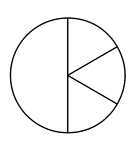### One Half and Three Sixths of a Pie Fraction

A circle subdivided into one half and three sixths.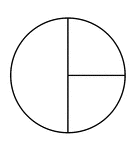### One Half and Two Quarters of a Pie Fraction

A circle subdivided into one half and two quarters.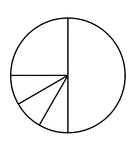### One Quarter, Three Twelfths, and One Half of a Pie Fraction

A circle subdivided into one quarter, three twelfths, and one half.### One Quarter, Two Eighths, and One Half of a Pie Fraction

A circle subdivided into one quarter, two eighths, and one half.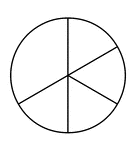### One Third and Four Sixths of a Pie Fraction

A circle subdivided into one third and four sixths.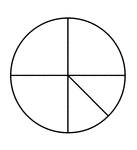### Three Quarters and Two Eighths of a Pie Fraction

A circle subdivided into three quarters and two eighths.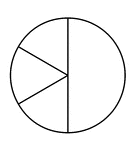### Three Sixths and One Half of a Pie Fraction

A circle subdivided into three sixths and one half.### Two Quarters and One Half of a Pie Fraction

A circle subdivided into two quarters and one half.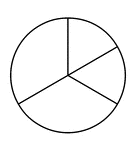### Two Thirds and Two Sixths of a Pie Fraction

A circle subdivided into two thirds and two sixths.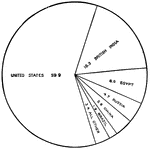### Pie Graph

A pie graph of "Proportion of World's Supply of Cotton contributed by each country" labeled with country…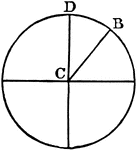### Sector

"In geometry: (a) A plane figure inclosed between the arc of a circle, ellipse, or other central curve…